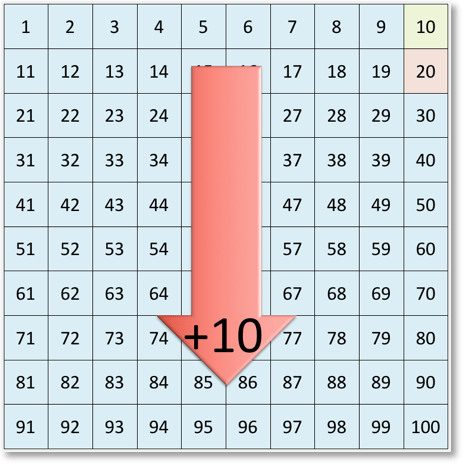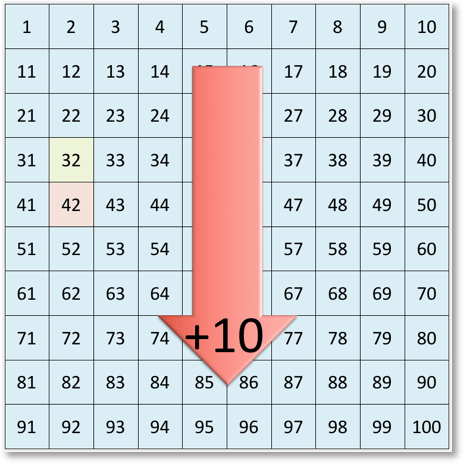# Number Grid: Adding and Subtracting Ten

Adding and Subtracting Ten: Video Lesson – Maths with Mum Download the Number Grid to 100 below:

Supporting Lessons

## How to Add or Subtract 10

If we choose a number on the number grid and move one place down, we are adding 10.

For example:If we begin with 10 and move one place down the number grid, we have added 10.

10 + 10 = 20.If we begin with 32 and move one place down the number grid, we have added 10.

32 + 10 = 42.

If we choose a number on the number grid and move one place up, we are subtracting 10.

For example:If we begin with 50 and move one place up the number grid, we have subtracted 10.

50 – 10 = 40.If we begin with 94 and move one place up the number grid, we have subtracted 10.

94 – 10 = 84.